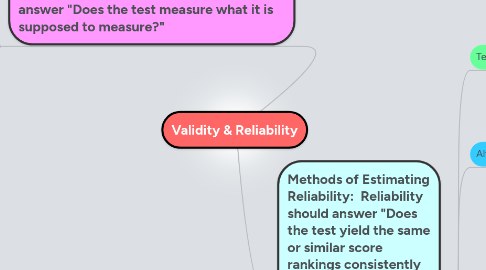# Validity & Reliability

Just an initial demo map, so that you don't start with an empty map list ...

Get Started. It's FreeValidity & Reliability## 1. Types of Validity Evidence: Validity should answer "Does the test measure what it is supposed to measure?"

### 1.1. Content

1.1.1. Reviews test questions to check if they go along with the material that is supposed to be covered by the test

1.1.2. Should answer the question "Does the test measure the instructional objectives?"

1.1.3. Can show if a test with high-stakes content is aligned with state standards

### 1.2. Criterion-related

1.2.1. Scores are compared to an outside measure

1.2.2. Two types

1.2.2.1. Concurrent

1.2.2.1.1. Determined by giving a new test and an established test, then finding the correlation between the two sets of scores

1.2.2.1.2. Can be a good way to estimate the strength of a new test

1.2.2.2. Predictive

1.2.2.2.1. Useful for aptitude tests, ex.: the SAT-II

1.2.2.2.2. How well the test predicts some future behavior of the test takers

1.2.3. Generates a numeric value

### 1.3. Construct

1.3.1. Information that shows if results from a test match up with what is expected

1.3.2. Results are compared to a theory or idea of how they should turn out

## 2. Methods of Estimating Reliability: Reliability should answer "Does the test yield the same or similar score rankings consistently with all other factors being equal?"

### 2.1. Test-Retest/Stability

2.1.1. A test is administered twice and a relationship is between the two sets of scores is looked at

2.1.1.1. A high correlation denotes a strong reliability

2.1.2. The time period between tests tends to affect the reliability coefficient

### 2.2. Alternate Forms/Equivalence

2.2.1. Students take two equal formats of a test and the relationship between the 2 sets of scores is calculated

2.2.2. It takes away the issue of memory, practice, study, etc. in the test-retest estimates

2.2.3. The two forms of the test need to be given under the same scenarios as close as possible

### 2.3. Internal Consistency

2.3.1. Can be used if the test covers a single concept

2.3.2. 2 Methods

2.3.2.1. Split-half (or odd-even)

2.3.2.1.1. Each question/item on the test is put with one half or the other half (like odd #'s and even #'s)

2.3.2.1.2. Each half is scored and the correlation between the two is calculated

2.3.2.2. Kuder-Richardson

2.3.2.2.1. Measures how similar items on one test are to another form of an equivalent test

2.3.3. Good because only one test is given; you don't have to make multiple forms of tests

2.3.4. Most widely used method of estimating the reliability of tests

2.3.5. Can underestimate the actual reliability of the full test